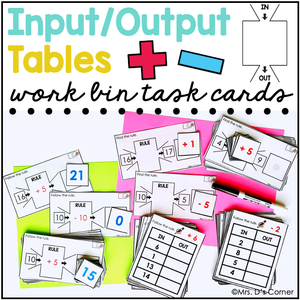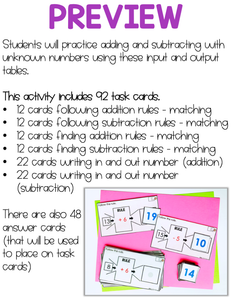# Input Output Tables Task Cards | Centers for Special Ed

Regular price
\$4.00
Sale price
\$4.00
Unit price
per
Shipping calculated at checkout.

INPUT OUTPUT TABLES WORK BIN TASK CARDS | Students will practice finding unknown numbers using addition and subtraction with these input and output table task cards.

Students will follow an addition or subtraction rule given, find the addition or subtraction rule, and apply an addition or subtraction rule to complete an in and out table.

What is included?

What skills are practiced?

Follow subtraction rules - 12 cards - match answer to input/output diagram following a given rule

Find addition rules - 12 cards - match answer to input/output diagram where in and out number is given

Find subtraction rules - 12 cards - match answer to input/output diagram where in and out number is given

Follow addition rule to fill in table - 22 cards, 11 each - fill in missing in and out numbers on an input/output table

Follow subtraction rule to fill in table - 22 cards, 11 each - fill in missing in and out numbers on an input/output table

Connect with me:

Don't forget about the green ★ to follow my store to get notifications of new resources and freebies!

Thanks for Looking and Happy Teaching!